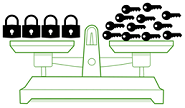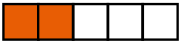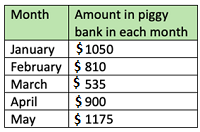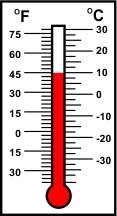# CREST Mathematics Olympiad Class 3 Sample Papers

REGISTER NOW

## Syllabus:

Section 1: Numerals, Number Names and Number Sense (4-digit numbers), Computation Operations, Fractions, Length, Weight, Capacity, Temperature, Time, Money, Geometry, Data Handling.

Achievers Section: Higher Order Thinking Questions - Syllabus as per Section 1

 Q.1 Using the given figure, find the weight of each lock if the weight of 3 keys is 1 kg:Q.2 Sandhya has only Rs. 120 in her purse. She wants to buy a crayon set and a sketch pen set costing Rs. 80 and Rs. 170, respectively. How much more money does she need?
 Q.3 Identify the value of X - Y: 1 X 5 2× 4 Y 0 0 8
 Q.4 Raju and Sonu have Rs. 120 and Rs. 135 each. Sohan has Rs. 35 more than the sum of the money of Raju and Sonu have taken together. How much money does Sohan have?
 Q.5 What fraction of the figure is unshaded?Q.6 Samay has 137 marbles with him. He gives 28 and 29 marbles to Raj and Rohit, respectively. How many marbles will be left with Samay?
 Q.7 Out of 28 chocolates, Vivek consumed one-fourth. How many chocolates are left?
 Q.8 The given table shows the amount of money that Harsha saved in her piggy bank each month. Find the total amount of money that she saved from January to May:Q.9 What is 5° more than the temperature shown in the thermometer given below?Q.10 The cost of a banana is Rs. 12. 28 dozens of bananas are kept in a sack. Find the cost of total bananas:

Sample PDF of CREST Mathematics Olympiad for Class 3: